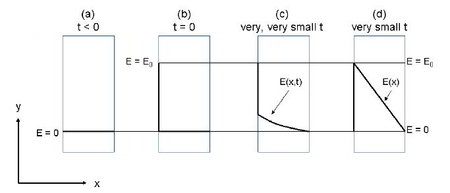Chemistry

# What is Transport of Electricity?

A flux of charges is induced when a driving force in the form of an electrical field is applied over a medium or a material that is able to conduct electrical charges. An electrical field has the unit of [V/m] corresponds to a voltage drop over a given length. In a wire, the electrical flux consists of moving electrons, and the flux multiplied by the cross-sectional area of the wire corresponds to the current measured in amperes [A]. The build-up of a voltage profile in a tin plate is sketched in below Figure.(a) The voltage on both sides of the tin plate is zero. (b) At t = 0 the voltage on the left side of the tin plate is increased to E0 while the voltage on the right side is kept a zero. (c) Electrical charges (electrons) start to flow through the tin plate because they are in an electrical field. At very, very small values of t, the voltage in the tin plate is a function of both time and distance x. (d) Very fast after the voltage on the left side is increased, steady state is reached and a linear voltage profile in the tin plate is established. Thus at steady state, the voltage is only a function of the distance x in the tin plate.

Initially, the voltage (energy pr. charge) is zero on both sides of the tin plate. No charges will then migrate through the tin plate. At time t= 0 the voltage on the left side is suddenly raised to E0 at which it is held constant. Charges (electrons) start to migrate through the tin plate because of the voltage difference. The voltage on the right side is kept at zero. The voltage thus increases in the plate as a function of the distance x and the time 1. Because tin is an electrical conductor, a steady state linear voltage profile through the plate is reached very fast, and thus the voltage is only a function of the distance x inside the tin plate after a very short period of time.At steady state, the Transport of Electricity flux (current density) through the membrane is given by the flux equation. which is called Ohm’s law:

Je = −σ·(dE / dx)

The flux ʃe is the flux of charges in the direction x through the tin plate. The gradient dE/dx, which is the voltage gradient inside the tin plate, is the driving force and is often called the electrical field. The electrical field can be explained as the effect that is produced by an electrical charge that exerts a force on charged objects in the field. The conductance σ of the tin plate is inversely proportional to the electrical resistivity of the tin plate. The resistivity tells how much the material or medium opposes the flow of the electrical current.

For a typical metal, the resistivity normally increases linearly with the temperature which means that the electrical conductance decreases with the temperature.